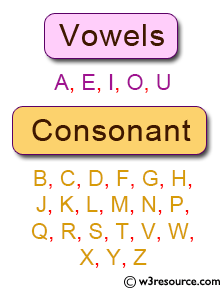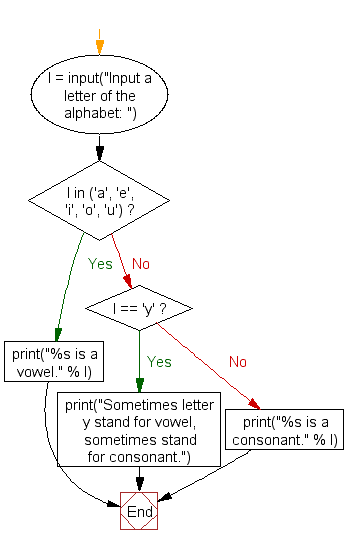﻿ Python Exercise: Check whether an alphabet is a vowel or consonant - w3resource# Python Exercise: Check whether an alphabet is a vowel or consonant

## Python Conditional: Exercise - 32 with Solution

Write a Python program to check whether an alphabet is a vowel or consonant.

Pictorial Presentation:Sample Solution:

Python Code:

``````l = input("Input a letter of the alphabet: ")

if l in ('a', 'e', 'i', 'o', 'u'):
print("%s is a vowel." % l)
elif l == 'y':
print("Sometimes letter y stand for vowel, sometimes stand for consonant.")
else:
print("%s is a consonant." % l)
```
```

Sample Output:

```Input a letter of the alphabet: u
u is a vowel.
```

Flowchart:Python Code Editor:

## Visualize Python code execution:

The following tool visualize what the computer is doing step-by-step as it executes the said program:

Have another way to solve this solution? Contribute your code (and comments) through Disqus.

What is the difficulty level of this exercise?

Test your Python skills with w3resource's quiz

﻿

## Python: Tips of the Day

Returns a list with n elements removed from the beginning

Example:

```def tips_take(itr, n = 1):
return itr[:n]
print(tips_take([1, 2, 3], 5))
print(tips_take([1, 2, 3], 0))
```

Output:

```[1, 2, 3]
[]
```